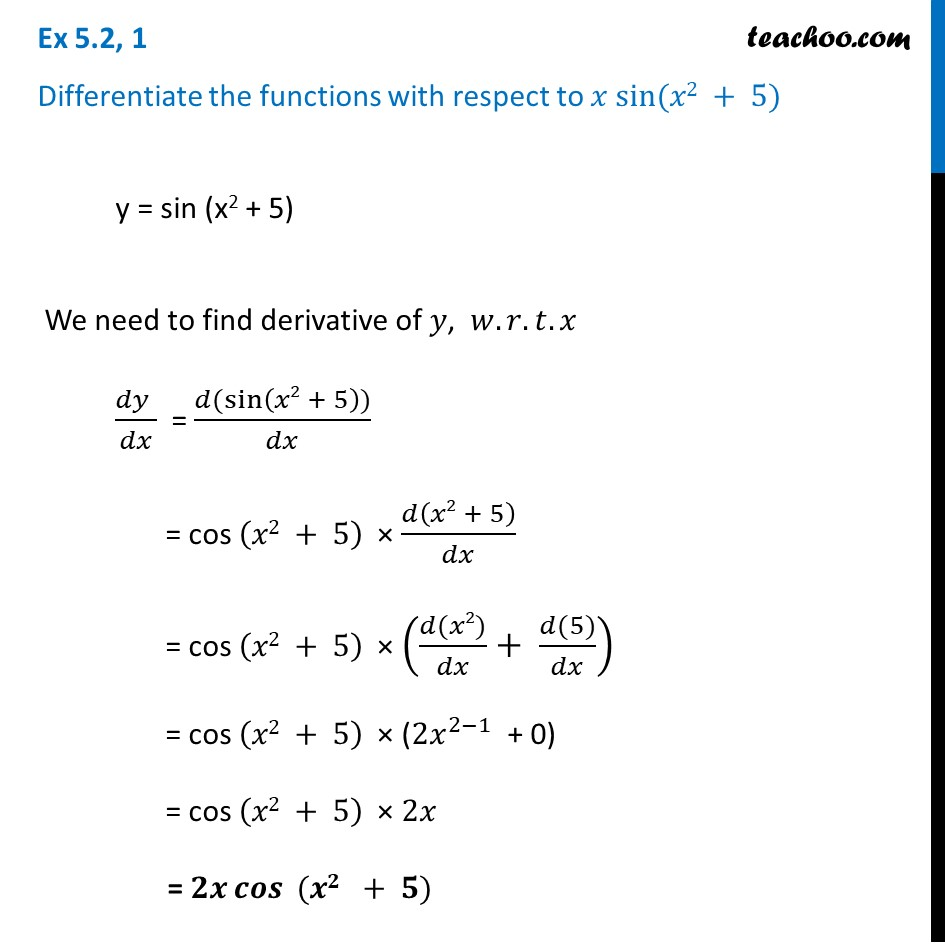Finding derivative of a function by chain rule

Chapter 5 Class 12 Continuity and Differentiability (Term 1)
Concept wise### Transcript

Ex 5.2, 1 Differentiate the functions with respect to 𝑥 sin⁡(𝑥2 + 5) y = sin (x2 + 5) We need to find derivative of 𝑦, 𝑤.𝑟.𝑡.𝑥 (𝑑𝑦 )/𝑑𝑥 = (𝑑(sin⁡(𝑥2 + 5)))/𝑑𝑥 = cos (𝑥2 + 5) × 𝑑(𝑥2 + 5)/𝑑𝑥 = cos (𝑥2 + 5) × ((𝑑(𝑥2))/𝑑𝑥+ (𝑑(5))/𝑑𝑥) = cos (𝑥2 + 5) × (〖2𝑥〗^(2−1) + 0) = cos (𝑥2 + 5) × 2𝑥 = 𝟐𝒙 𝒄𝒐𝒔⁡〖 (𝒙𝟐 + 𝟓)〗